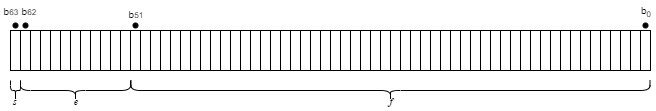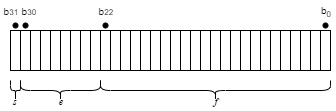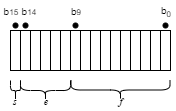## Floating-Point Numbers

### Floating-Point Numbers

Fixed-point numbers are limited in that they cannot simultaneously represent very large or very small numbers using a reasonable word size. This limitation can be overcome by using scientific notation. With scientific notation, you can dynamically place the binary point at a convenient location and use powers of the binary to keep track of that location. Thus, you can represent a range of very large and very small numbers with only a few digits.

You can represent any binary floating-point number in scientific notation form as f2e, where f is the fraction (or mantissa), `2` is the radix or base (binary in this case), and e is the exponent of the radix. The radix is always a positive number, while f and e can be positive or negative.

When performing arithmetic operations, floating-point hardware must take into account that the sign, exponent, and fraction are all encoded within the same binary word. This results in complex logic circuits when compared with the circuits for binary fixed-point operations.

The Fixed-Point Designer™ software supports single-precision and double-precision floating-point numbers as defined by the IEEE® Standard 754.

### Scientific Notation

A direct analogy exists between scientific notation and radix point notation. For example, scientific notation using five decimal digits for the fraction would take the form

`$±d.dddd×{10}^{p}=±ddddd.0×{10}^{p-4}=±0.ddddd×{10}^{p+1},$`

where $d=0,...,9$ and p is an integer of unrestricted range.

Radix point notation using five bits for the fraction is the same except for the number base

`$±b.bbbb×{2}^{q}=±bbbbb.0×{2}^{q-4}=±0.bbbbb×{2}^{q+1},$`

where $b=0,1$ and q is an integer of unrestricted range.

For fixed-point numbers, the exponent is fixed but there is no reason why the binary point must be contiguous with the fraction. For more information, see Binary Point Interpretation.

### IEEE 754 Standard for Floating-Point Numbers

The IEEE Standard 754 has been widely adopted, and is used with virtually all floating-point processors and arithmetic coprocessors, with the notable exception of many DSP floating-point processors.

This standard specifies several floating-point number formats, of which singles and doubles are the most widely used. Each format contains three components: a sign bit, a fraction field, and an exponent field.

#### The Sign Bit

IEEE floating-point numbers use sign/magnitude representation, where the sign bit is explicitly included in the word. Using sign/magnitude representation, a sign bit of 0 represents a positive number and a sign bit of 1 represents a negative number. This is in contrast to the two's complement representation preferred for signed fixed-point numbers.

#### The Fraction Field

Floating-point numbers can be represented in many different ways by shifting the number to the left or right of the binary point and decreasing or increasing the exponent of the binary by a corresponding amount.

To simplify operations on floating-point numbers, they are normalized in the IEEE format. A normalized binary number has a fraction of the form 1.f, where f has a fixed size for a given data type. Since the leftmost fraction bit is always a 1, it is unnecessary to store this bit and it is therefore implicit (or hidden). Thus, an n-bit fraction stores an n+1-bit number. The IEEE format also supports Denormalized Numbers, which have a fraction of the form 0.f.

#### The Exponent Field

In the IEEE format, exponent representations are biased. This means a fixed value, the bias, is subtracted from the exponent field to get the true exponent value. For example, if the exponent field is 8 bits, then the numbers 0 through 255 are represented, and there is a bias of 127. Note that some values of the exponent are reserved for flagging `Inf` (infinity), `NaN` (not-a-number), and denormalized numbers, so the true exponent values range from -126 to 127. See Inf and NaN for more information.

#### Double-Precision Format

The IEEE double-precision floating-point format is a 64-bit word divided into a 1-bit sign indicator s, an 11-bit biased exponent e, and a 52-bit fraction f.The relationship between double-precision format and the representation of real numbers is given by

#### Single-Precision Format

The IEEE single-precision floating-point format is a 32-bit word divided into a 1-bit sign indicator s, an 8-bit biased exponent e, and a 23-bit fraction f.The relationship between single-precision format and the representation of real numbers is given by

#### Half-Precision Format

The IEEE half-precision floating-point format is a 16-bit word divided into a 1-bit sign indicator s, a 5-bit biased exponent e, and a 10-bit fraction f.Half-precision numbers are supported only in MATLAB®. For more information, see `half`.

### Range and Precision

The range of a number gives the limits of the representation. The precision gives the distance between successive numbers in the representation. The range and precision of an IEEE floating-point number depends on the specific format.

#### Range

The range of representable numbers for an IEEE floating-point number with f bits allocated for the fraction, e bits allocated for the exponent, and the bias of e given by bias = 2(e−1)−1 is given below.where

• Normalized positive numbers are defined within the range 2(1−bias) to (2−2f)2bias.

• Normalized negative numbers are defined within the range −2(1−bias) to −(2−2f)2bias.

• Positive numbers greater than (2−2f)2bias and negative numbers less than −(2−2f)2bias are overflows.

• Positive numbers less than 2(1−bias) and negative numbers greater than −2(1−bias) are either underflows or denormalized numbers.

• Zero is given by a special bit pattern, where e = 0 and f = 0.

Overflows and underflows result from exceptional arithmetic conditions. Floating-point numbers outside the defined range are always mapped to ±`Inf`.

### Note

You can use the MATLAB commands `realmin` and `realmax` to determine the dynamic range of double-precision floating-point values for your computer.

#### Precision

A floating-point number is only an approximation of the “true” value because of a finite word size. Therefore, it is important to have an understanding of the precision (or accuracy) of a floating-point result. A value v with an accuracy q is specified by v ± q. For IEEE floating-point numbers,

v = (−1)s(2ebias)(1.f)

and

q = 2f × 2ebias

Thus, the precision is associated with the number of bits in the fraction field.

### Note

In the MATLAB software, floating-point relative accuracy is given by the command `eps`, which returns the distance from 1.0 to the next larger floating-point number. For a computer that supports the IEEE Standard 754, `eps` = 2−52 or 2.22045 · 10-16.

#### Floating-Point Data Type Parameters

The range, bias, and precision for supported floating-point data types are given in the table below.

Data Type

Low Limit

High Limit

Exponent Bias

Precision

Half (MATLAB only)

2−14 ≈ 10−4

216 ≈ 105

15

2−10 ≈ 10 · 10−4

Single

2−126 ≈ 10−38

2128 ≈ 3 · 1038

127

2−23 ≈ 10−7

Double

2−1022 ≈ 2 · 10−308

21024 ≈ 2 · 10308

1023

2−52 ≈ 10−16

Because floating-point numbers are represented using sign/magnitude, there are two representations of zero, one positive and one negative. For both representations e = 0 and f.0 = 0.0.

### Exceptional Arithmetic

The IEEE Standard 754 specifies practices and procedures so that predictable results are produced independently of the hardware platform. Denormalized numbers, `Inf`, and `NaN` are defined to deal with exceptional arithmetic (underflow and overflow).

If an underflow or overflow is handled as `Inf` or `NaN`, then significant processor overhead is required to deal with this exception. Although the IEEE Standard 754 specifies practices and procedures to deal with exceptional arithmetic conditions in a consistent manner, microprocessor manufacturers might handle these conditions in ways that depart from the standard.

#### Denormalized Numbers

Denormalized numbers are used to handle cases of exponent underflow. When the exponent of the result is too small (i.e., a negative exponent with too large a magnitude), the result is denormalized by right-shifting the fraction and leaving the exponent at its minimum value. The use of denormalized numbers is also referred to as gradual underflow. Without denormalized numbers, the gap between the smallest representable nonzero number and zero is much wider than the gap between the smallest representable nonzero number and the next larger number. Gradual underflow fills that gap and reduces the impact of exponent underflow to a level comparable with round-off among the normalized numbers. Denormalized numbers provide extended range for small numbers at the expense of precision.

#### Inf

Arithmetic involving `Inf` (infinity) is treated as the limiting case of real arithmetic, with infinite values defined as those outside the range of representable numbers, or −∞ ≤ (representable numbers) < ∞. With the exception of the special cases discussed below (`NaN`), any arithmetic operation involving `Inf` yields `Inf`. `Inf` is represented by the largest biased exponent allowed by the format and a fraction of zero.

#### NaN

A `NaN` (not-a-number) is a symbolic entity encoded in floating-point format. There are two types of `NaN`: signaling and quiet. A signaling `NaN` signals an invalid operation exception. A quiet `NaN` propagates through almost every arithmetic operation without signaling an exception. The following operations result in a `NaN`: ∞–∞, –∞+∞, 0×∞, 0/0, and ∞/∞.

Both signaling `NaN` and quiet `NaN` are represented by the largest biased exponent allowed by the format and a nonzero fraction. The bit pattern for a quiet `NaN` is given by 0.f, where the most significant bit in f must be a one. The bit pattern for a signaling `NaN` is given by 0.f, where the most significant bit in f must be zero and at least one of the remaining bits must be nonzero.

## Related Topics

##### SupportGet trial now